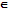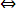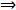Loading

# Define the orbit of a permutation

Publish On: 2019-05-17

# Ben joy

Total Post: 542

## Question: Define the orbit of a permutation

Reply On: 2013-10-07

# Mjay Jollay

Total Post: 0

## ANS: Define the orbit of a permutation

Let ƒ be any permutation on a set S. If a relation ~ is defined on S such that a ~ b ⟺ ƒ(n) (a) = b for some integer n and ∀ a, bS, we observe that the relation ‘~’ is

(i) Reflexive, because a ~ aƒ(n) (a) = I (a) = a ∀ aS.

(ii) Symmetric, because a ~ bƒ(n) (a) = b for some integer na = ƒ(n) (b)b – a for a, bS.

(iii) Transitive, because a ~ b and b ~ cƒ(n) (a) = b, ƒ(m) (b) = c for some integers n and m.ƒmn (a)) = ƒm (b) = cƒm+n (a) = c for some integer (m + n)a ~ c.

Thus above defined relation ~ is an equivalence relation on S and hence it partitions S into mutually disjoint classes. Each equivalence class determined by the above relation is called an orbit of ƒ.

#### Submit Your Answer

warning: Please Login To Submit Your Answer
Like Us On Facebook for All Latest Updates